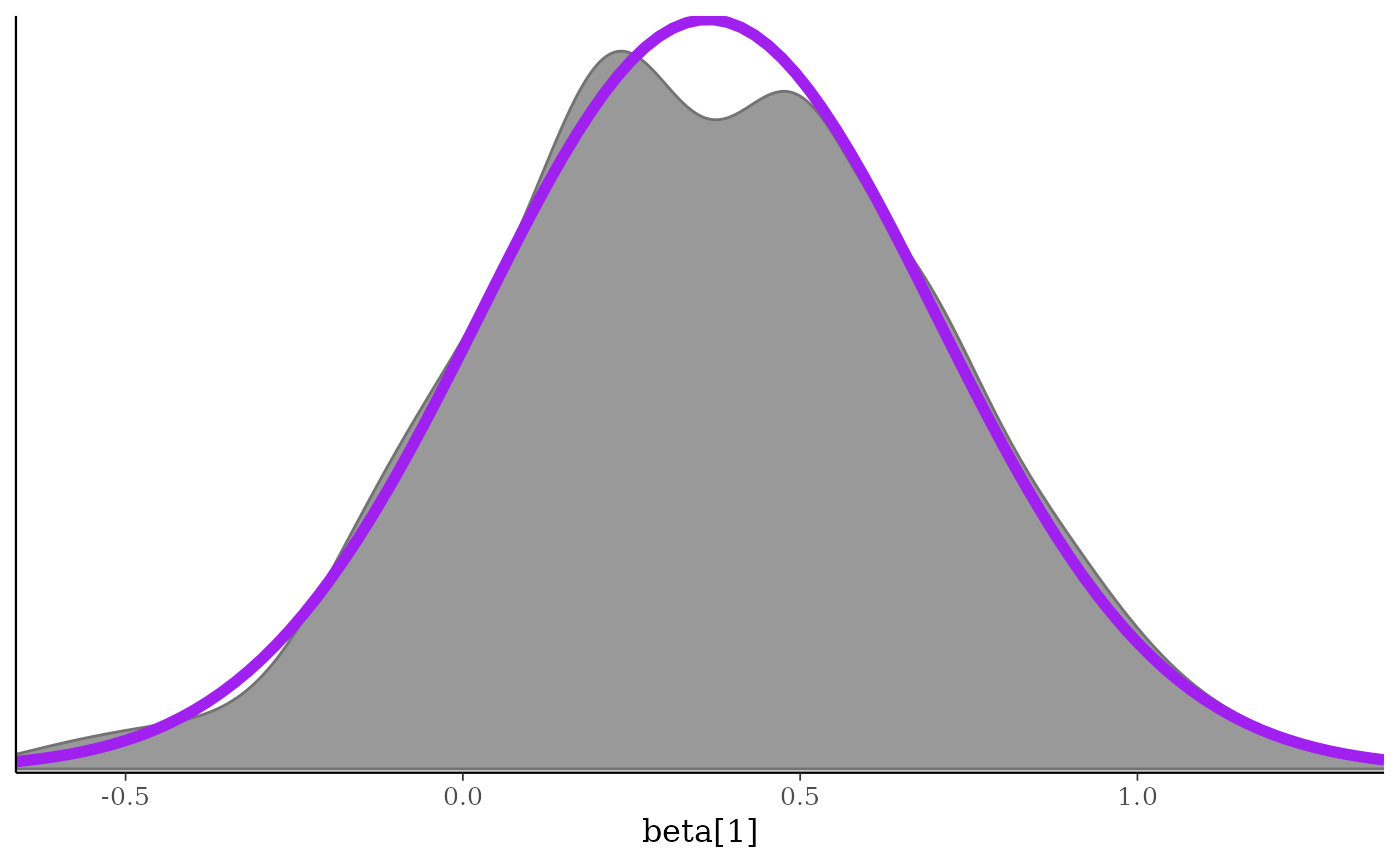Convenience functions for adding to (and changing details of) ggplot objects (many of the objects returned by bayesplot functions). See the Examples section, below.

vline_at(v, fun, ..., na.rm = TRUE)

hline_at(v, fun, ..., na.rm = TRUE)

vline_0(..., na.rm = TRUE)

hline_0(..., na.rm = TRUE)

abline_01(..., na.rm = TRUE)

lbub(p, med = TRUE)

legend_move(position = "right")

legend_none()

legend_text(...)

xaxis_title(on = TRUE, ...)

xaxis_text(on = TRUE, ...)

xaxis_ticks(on = TRUE, ...)

yaxis_title(on = TRUE, ...)

yaxis_text(on = TRUE, ...)

yaxis_ticks(on = TRUE, ...)

facet_text(on = TRUE, ...)

facet_bg(on = TRUE, ...)

panel_bg(on = TRUE, ...)

plot_bg(on = TRUE, ...)

grid_lines(color = "gray50", size = 0.2)

overlay_function(...)

## Arguments

v Either a numeric vector specifying the value(s) at which to draw the vertical or horizontal line(s), or an object of any type to use as the first argument to fun. A function, or the name of a function, that returns a numeric vector. For the various vline_, hline_, and abline_ functions, ... is passed to ggplot2::geom_vline(), ggplot2::geom_hline(), and ggplot2::geom_abline(), respectively, to control the appearance of the line(s). For functions ending in _bg, ... is passed to ggplot2::element_rect(). For functions ending in _text or _title, ... is passed to ggplot2::element_text(). For xaxis_ticks and yaxis_ticks, ... is passed to ggplot2::element_line(). For overlay_function, ... is passed to ggplot2::stat_function(). A logical scalar passed to the appropriate geom (e.g. ggplot2::geom_vline()). The default is TRUE. The probability mass (in [0,1]) to include in the interval. Should the median also be included in addition to the lower and upper bounds of the interval? The position of the legend. Either a numeric vector (of length 2) giving the relative coordinates (between 0 and 1) for the legend, or a string among "right", "left", "top", "bottom". Using position = "none" is also allowed and is equivalent to using legend_none(). For functions modifying ggplot theme elements, set on=FALSE to set the element to ggplot2::element_blank(). For example, facet text can be removed by adding facet_text(on=FALSE), or simply facet_text(FALSE) to a ggplot object. If on=TRUE (the default), then ... can be used to customize the appearance of the theme element. Passed to ggplot2::element_line().

## Value

A ggplot2 layer or ggplot2::theme() object that can be added to existing ggplot objects, like those created by many of the bayesplot plotting functions. See the Details section.

## Details

### Add vertical, horizontal, and diagonal lines to plots

• vline_at() and hline_at() return an object created by either ggplot2::geom_vline() or ggplot2::geom_hline() that can be added to a ggplot object to draw a vertical or horizontal line (at one or several values). If fun is missing then the lines are drawn at the values in v. If fun is specified then the lines are drawn at the values returned by fun(v).

• vline_0() and hline_0() are wrappers for vline_at() and hline_at() with v = 0 and fun missing.

• abline_01() is a wrapper for ggplot2::geom_abline() with the intercept set to 0 and the slope set to 1.

• lbub() returns a function that takes a single argument x and returns the lower and upper bounds (lb, ub) of the 100*p\ of x, as well as the median (if med=TRUE).

### Control appearance of facet strips

• facet_text() returns ggplot2 theme objects that can be added to an existing plot (ggplot object) to format the text in facet strips.

• facet_bg() can be added to a plot to change the background of the facet strips.

### Move legend, remove legend, or style the legend text

• legend_move() and legend_none() return a ggplot2 theme object that can be added to an existing plot (ggplot object) in order to change the position of the legend or remove it.

• legend_text() works much like facet_text() but for the legend.

### Control appearance of $$x$$-axis and $$y$$-axis features

• xaxis_title() and yaxis_title() return a ggplot2 theme object that can be added to an existing plot (ggplot object) in order to toggle or format the titles displayed on the x or y axis. (To change the titles themselves use ggplot2::labs().)

• xaxis_text() and yaxis_text() return a ggplot2 theme object that can be added to an existing plot (ggplot object) in order to toggle or format the text displayed on the x or y axis (e.g. tick labels).

• xaxis_ticks() and yaxis_ticks() return a ggplot2 theme object that can be added to an existing plot (ggplot object) to change the appearance of the axis tick marks.

### Customize plot background

• plot_bg() returns a ggplot2 theme object that can be added to an existing plot (ggplot object) to format the background of the entire plot.

• panel_bg() returns a ggplot2 theme object that can be added to an existing plot (ggplot object) to format the background of the just the plotting area.

• grid_lines() returns a ggplot2 theme object that can be added to an existing plot (ggplot object) to add grid lines to the plot background.

### Superimpose a function on an existing plot

• overlay_function() is a simple wrapper for ggplot2::stat_function() but with the inherit.aes argument fixed to FALSE. Fixing inherit.aes=FALSE will avoid potential errors due to the ggplot2::aes()thetic mapping used by certain bayesplot plotting functions.

## See also

theme_default() for the default ggplot theme used by bayesplot.

## Examples

color_scheme_set("gray")
x <- example_mcmc_draws(chains = 1)
dim(x)#>  250   4colnames(x)#>  "alpha"   "sigma"   "beta" "beta"

###################################
### vertical & horizontal lines ###
###################################
(p <- mcmc_intervals(x, regex_pars = "beta"))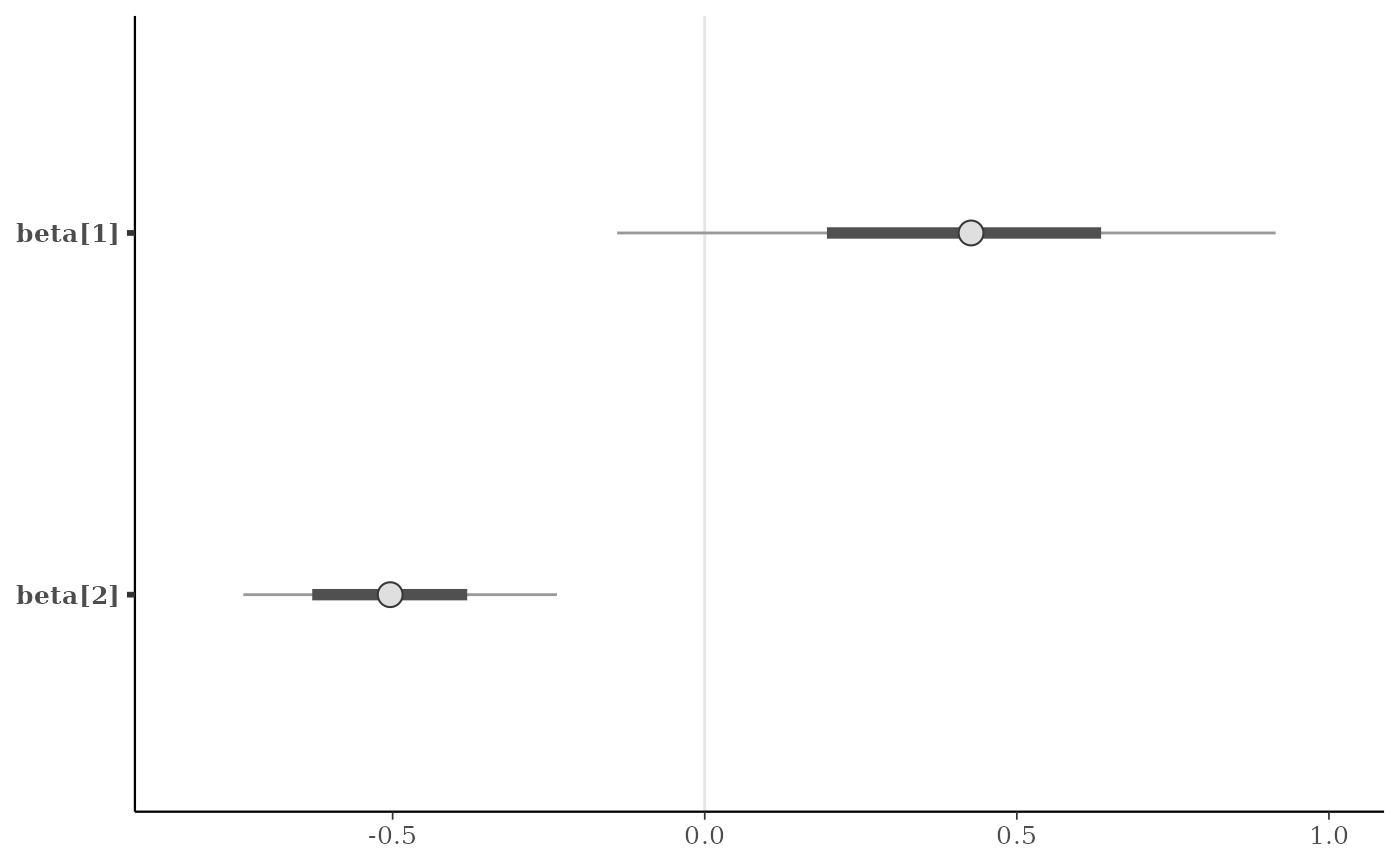# vertical line at zero (with some optional styling)
p + vline_0()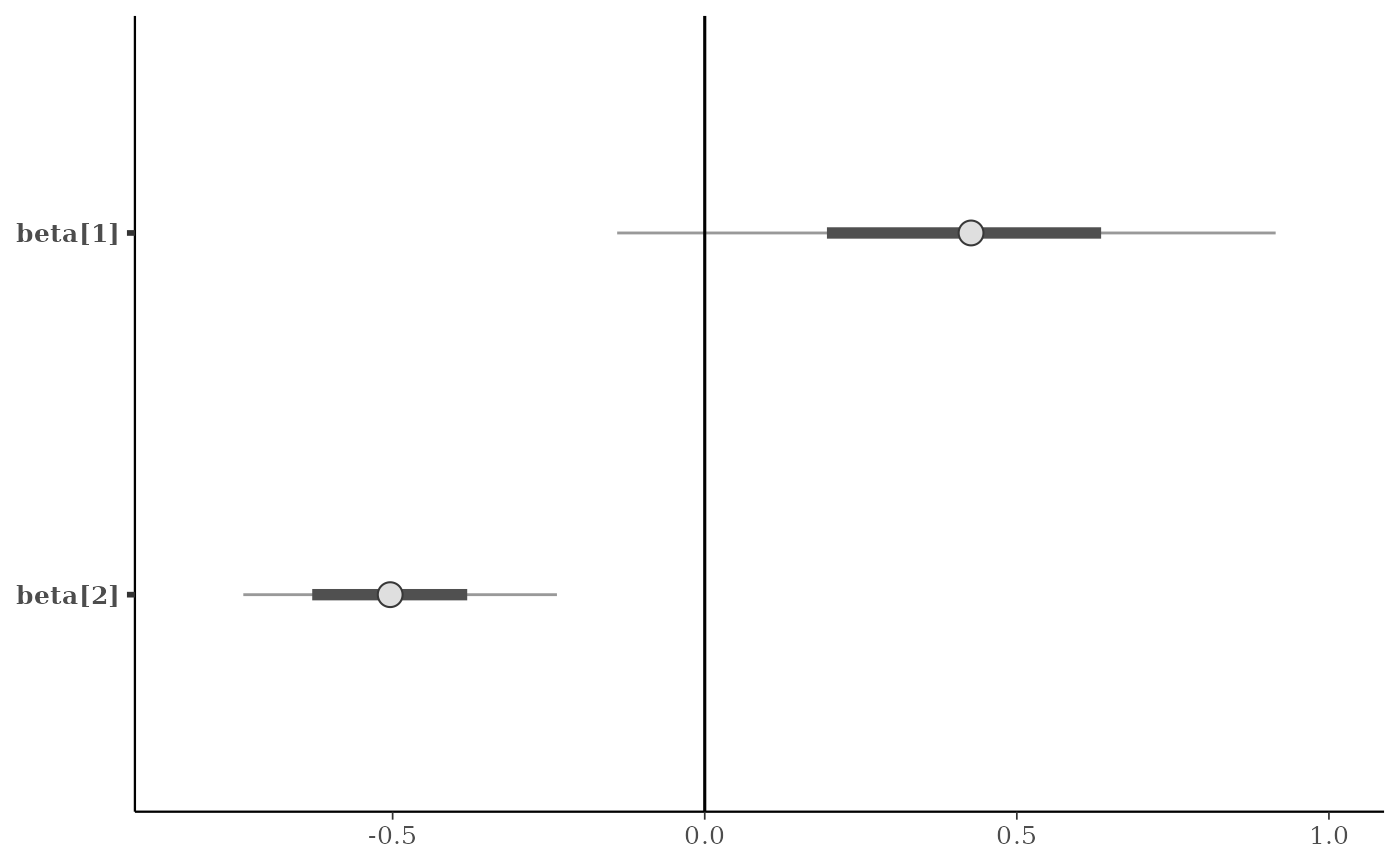p + vline_0(size = 0.25, color = "darkgray", linetype = 2)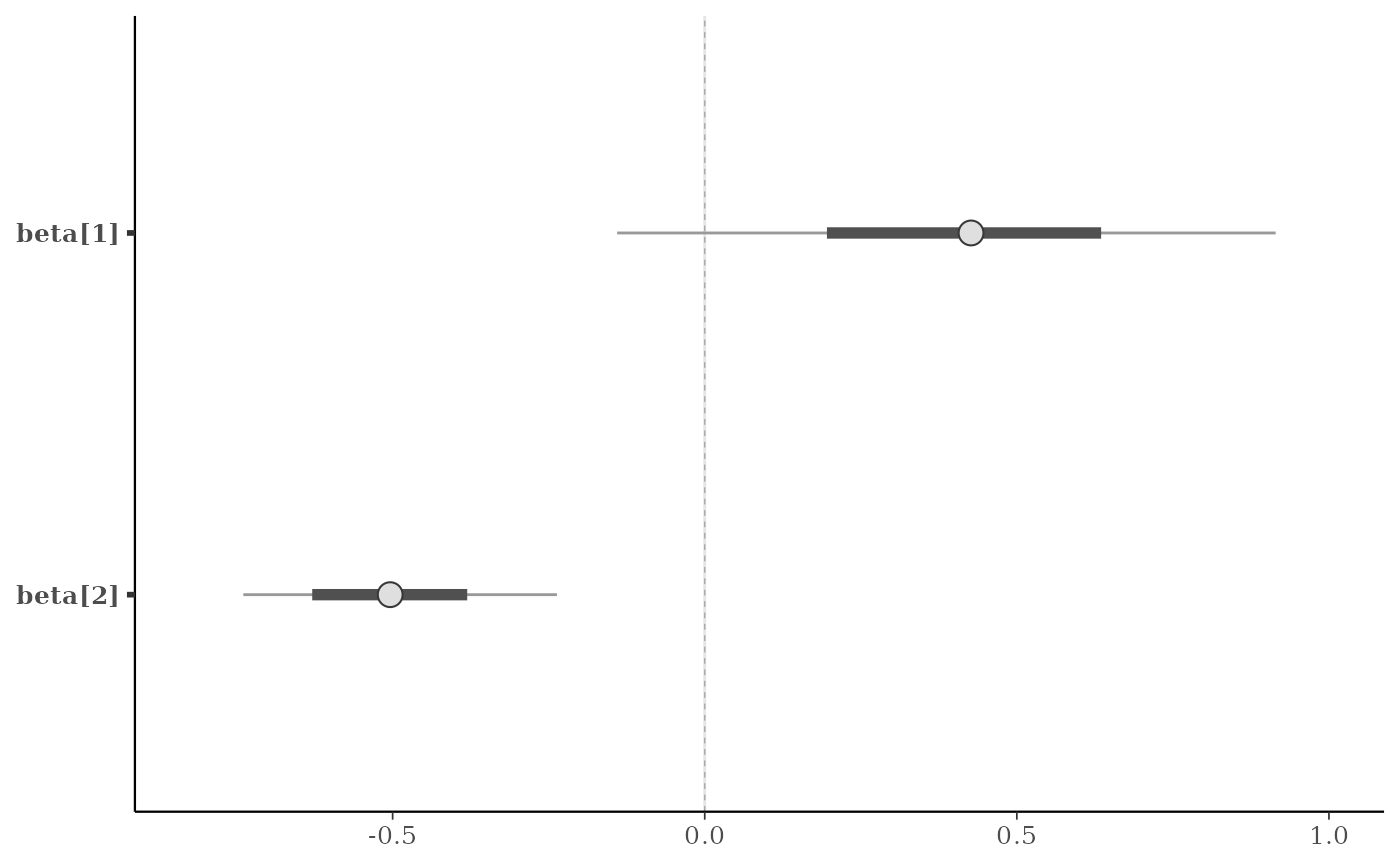# vertical line(s) at specified values
v <- c(-0.5, 0, 0.5)
p + vline_at(v, linetype = 3, size = 0.25)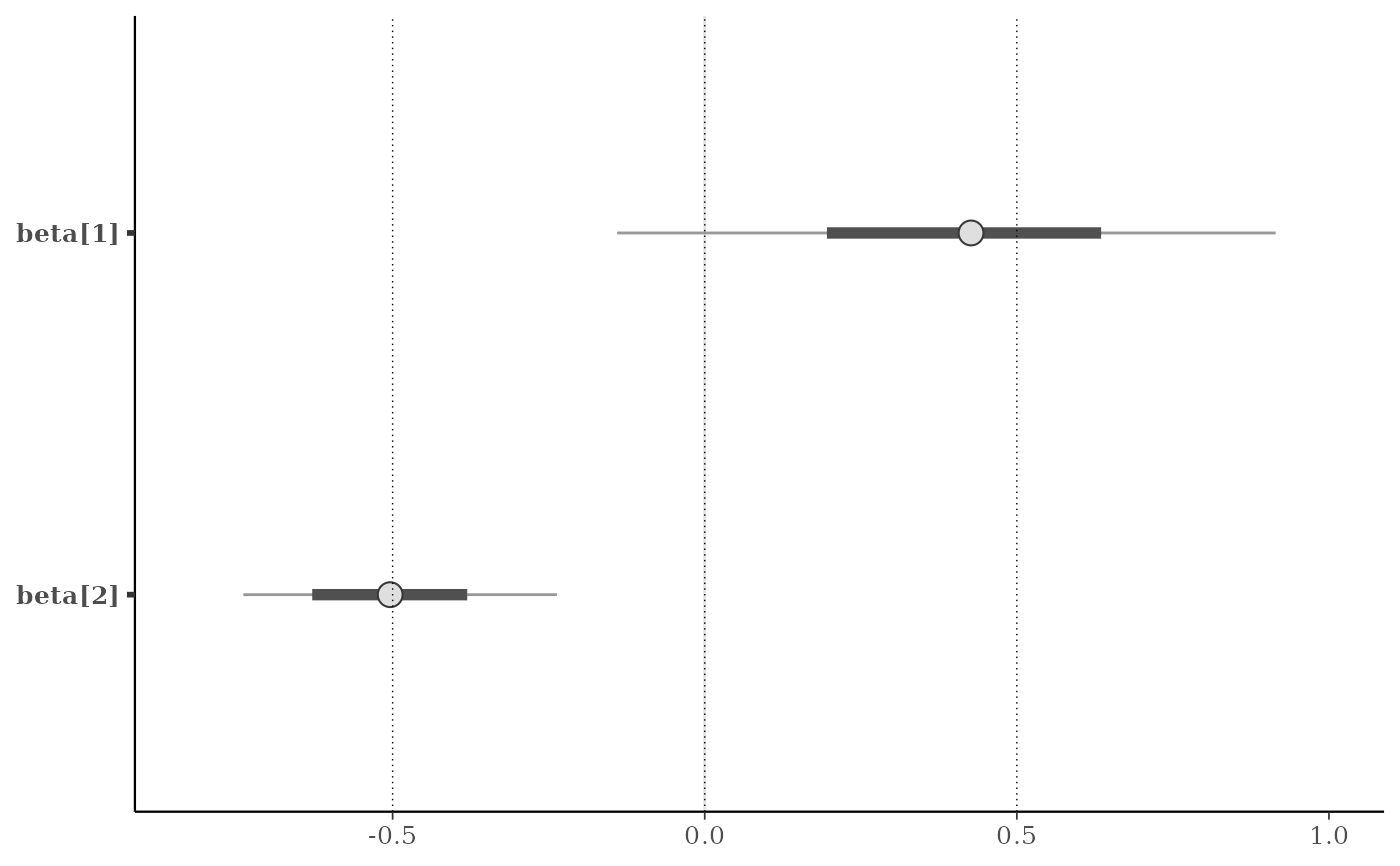my_lines <- vline_at(v, alpha = 0.25, size = 0.75 * c(1, 2, 1),
color = c("maroon", "skyblue", "violet"))
p + my_lines# \donttest{
# add vertical line(s) at computed values
# (three ways of getting lines at column means)
color_scheme_set("brightblue")
p <- mcmc_intervals(x, regex_pars = "beta")
p + vline_at(x[, 3:4], colMeans)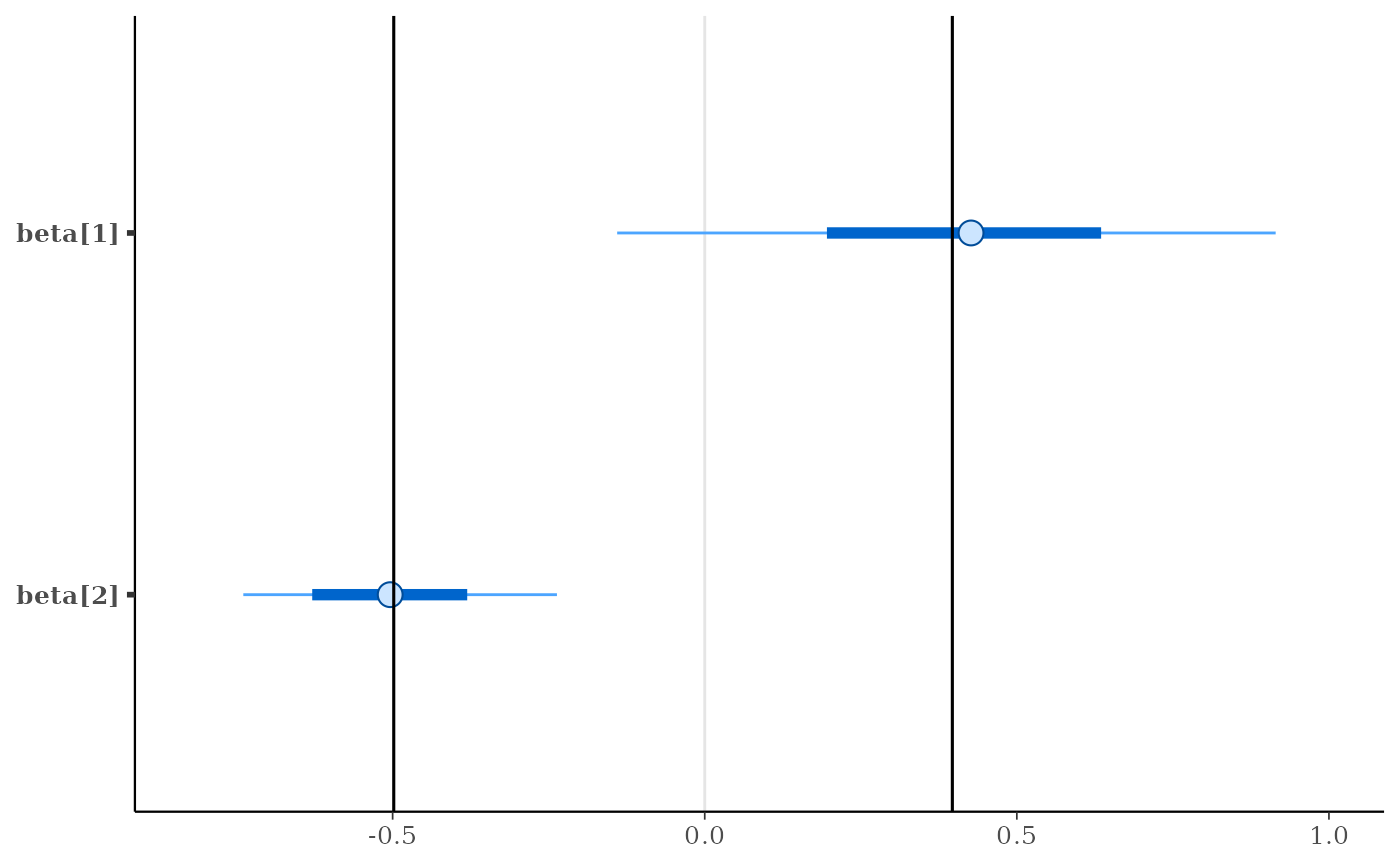p + vline_at(x[, 3:4], "colMeans", color = "darkgray",
lty = 2, size = 0.25)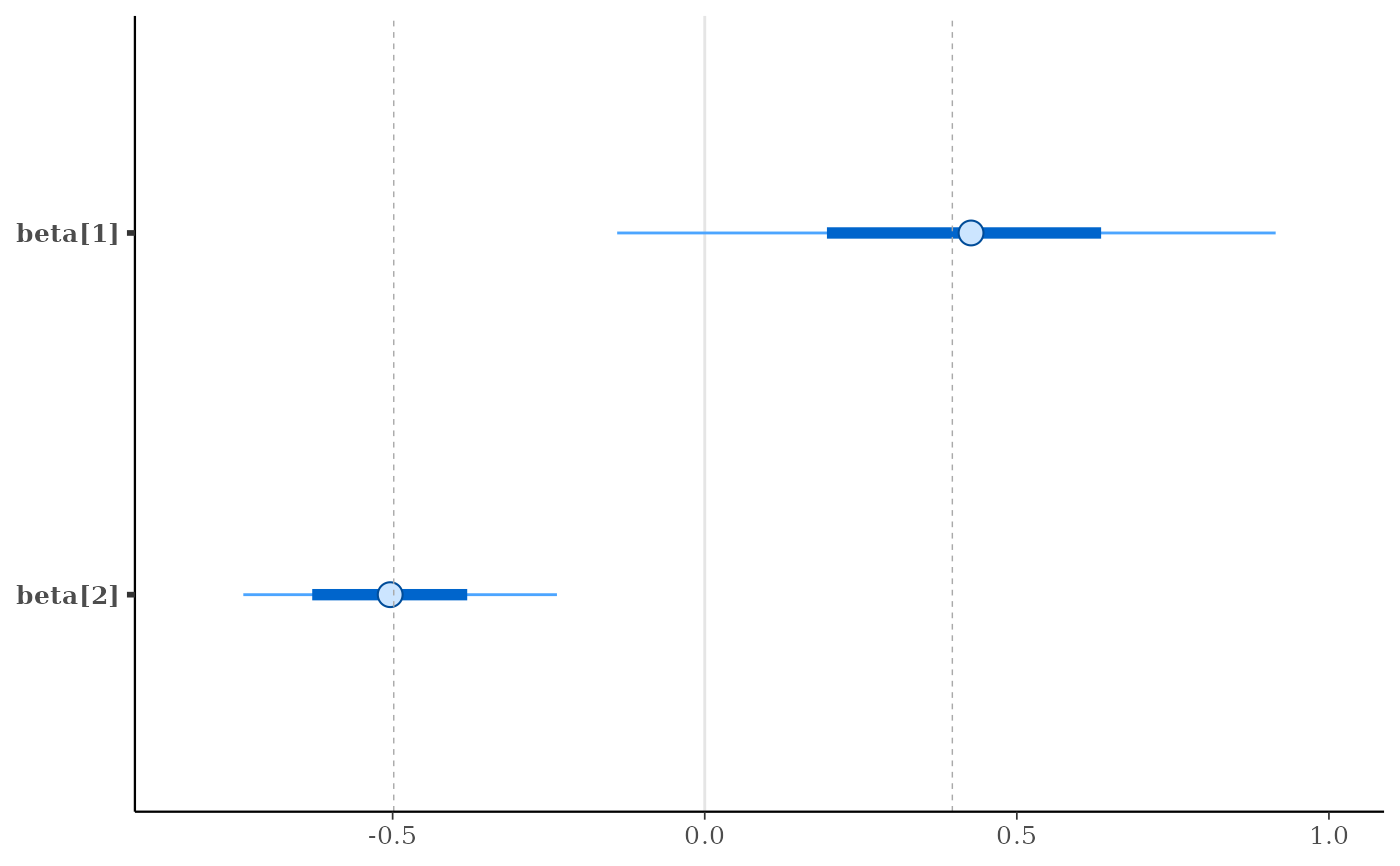p + vline_at(x[, 3:4], function(a) apply(a, 2, mean),
color = "orange",
size = 2, alpha = 0.1)# }

# using the lbub function to get interval lower and upper bounds (lb, ub)
color_scheme_set("pink")
parsed <- ggplot2::label_parsed
p2 <- mcmc_hist(x, pars = "beta", binwidth = 1/20,
facet_args = list(labeller = parsed))
(p2 <- p2 + facet_text(size = 16))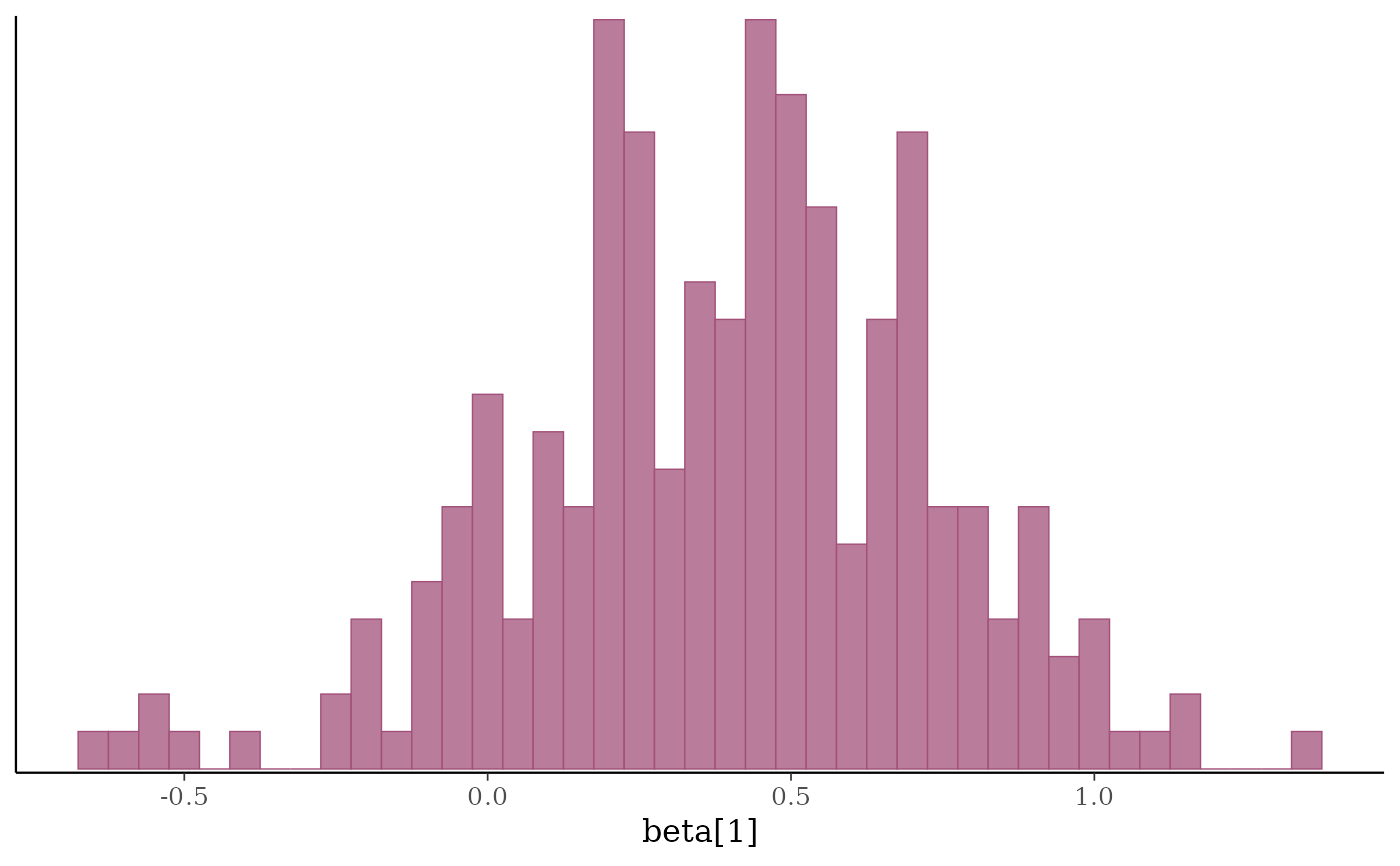b1 <- x[, "beta"]
p2 + vline_at(b1, fun = lbub(0.8), color = "gray20",
size = 2 * c(1,.5,1), alpha = 0.75)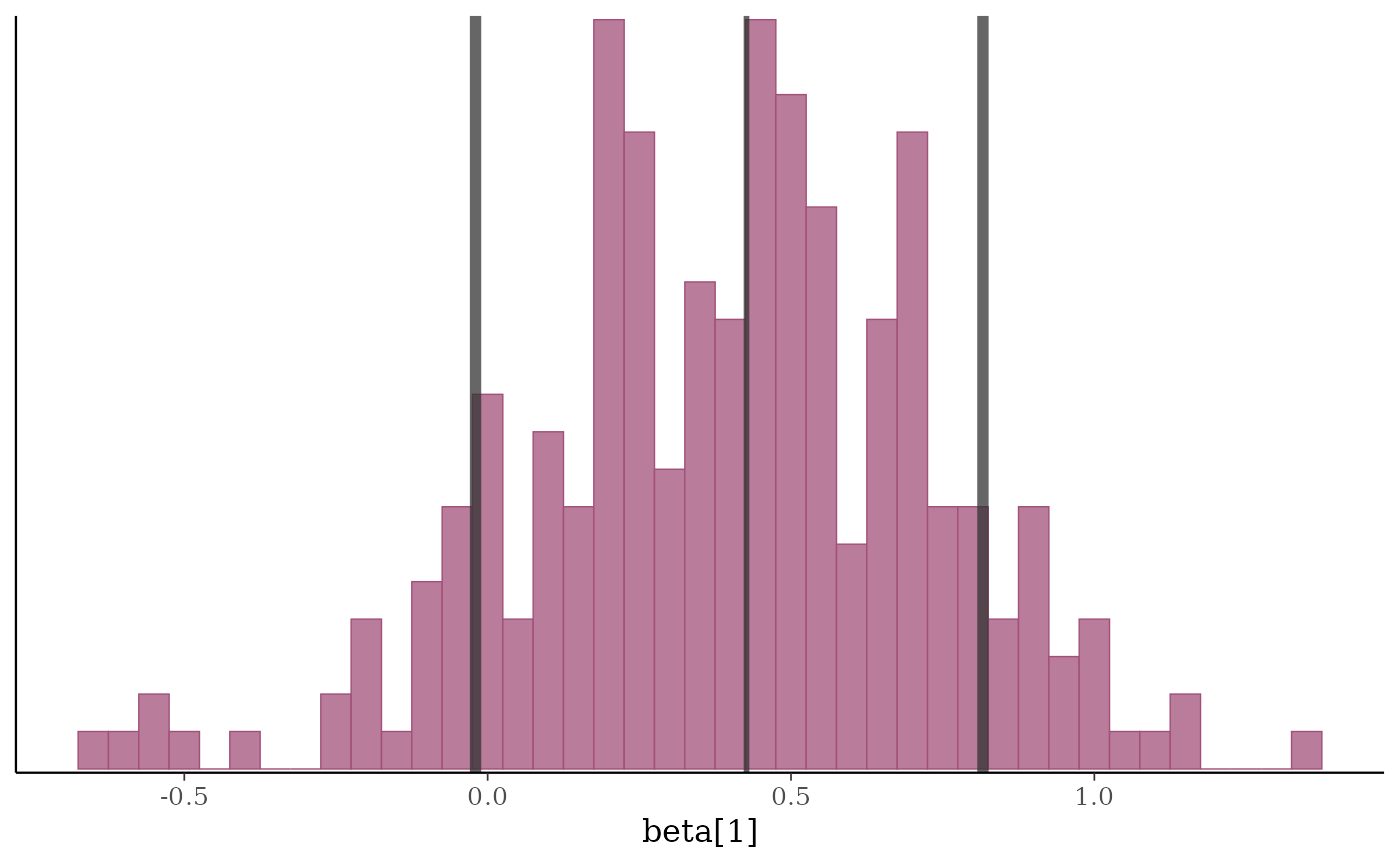p2 + vline_at(b1, lbub(0.8, med = FALSE), color = "gray20",
size = 2, alpha = 0.75)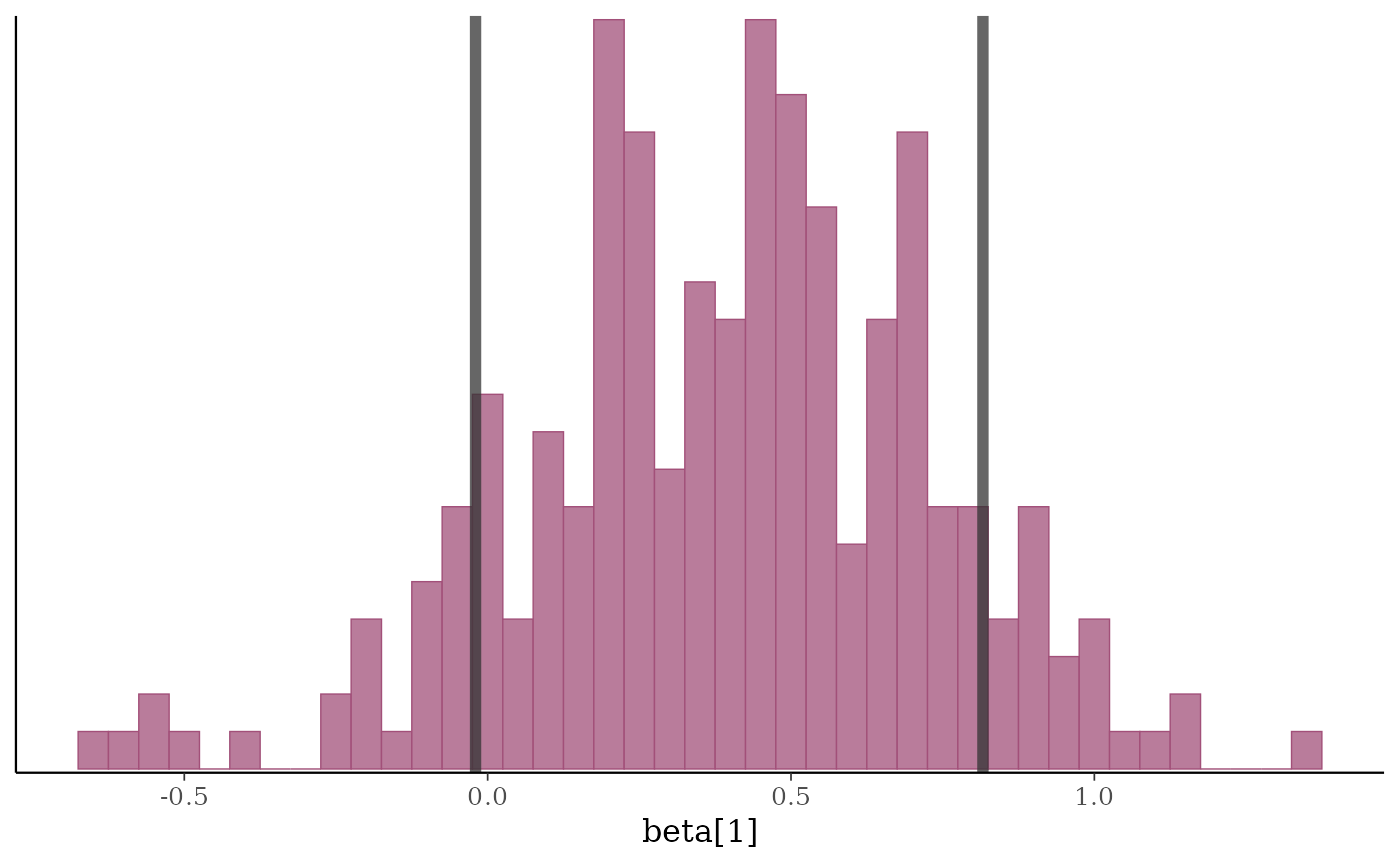##########################
### format axis titles ###
##########################
color_scheme_set("green")
y <- example_y_data()
yrep <- example_yrep_draws()
(p3 <- ppc_stat(y, yrep, stat = "median", binwidth = 1/4))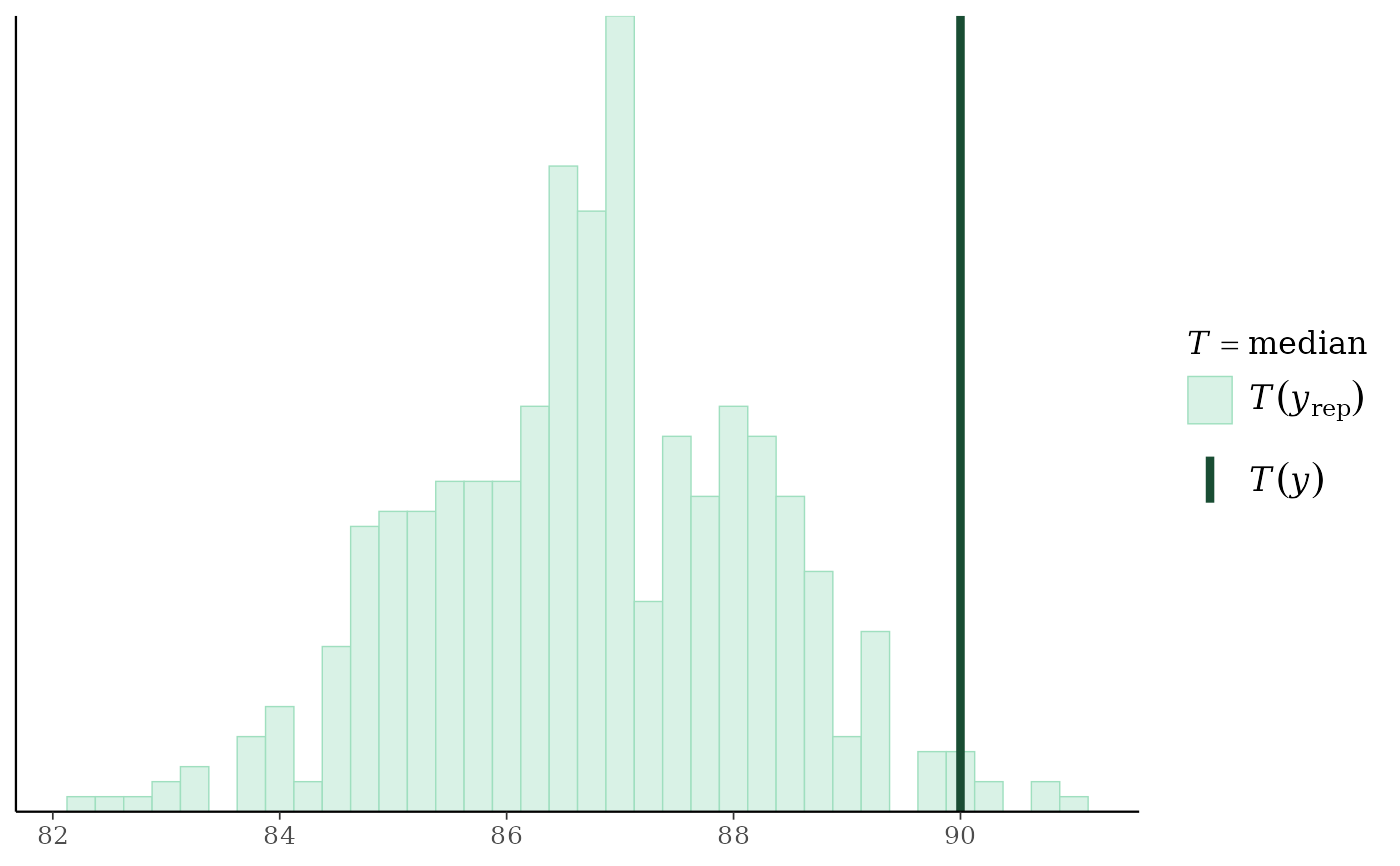# turn off the legend, turn on x-axis title
p3 +
legend_none() +
xaxis_title(size = 13, family = "sans") +
ggplot2::xlab(expression(italic(T(y)) == median(italic(y))))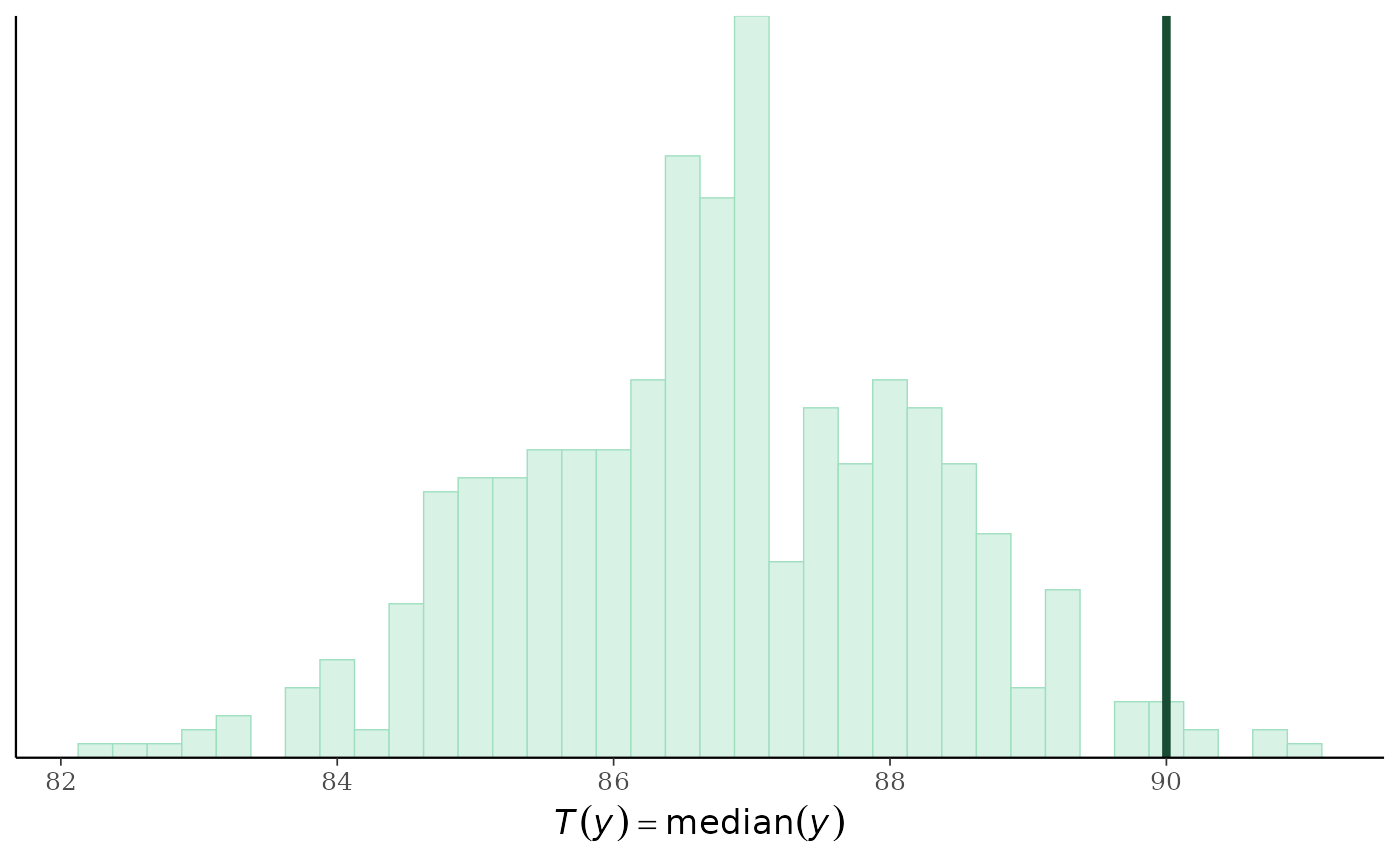################################
### format axis & facet text ###
################################
color_scheme_set("gray")
p4 <- mcmc_trace(example_mcmc_draws(), pars = c("alpha", "sigma"))

myfacets <-
facet_bg(fill = "gray30", color = NA) +
facet_text(face = "bold", color = "skyblue", size = 14)
p4 + myfacets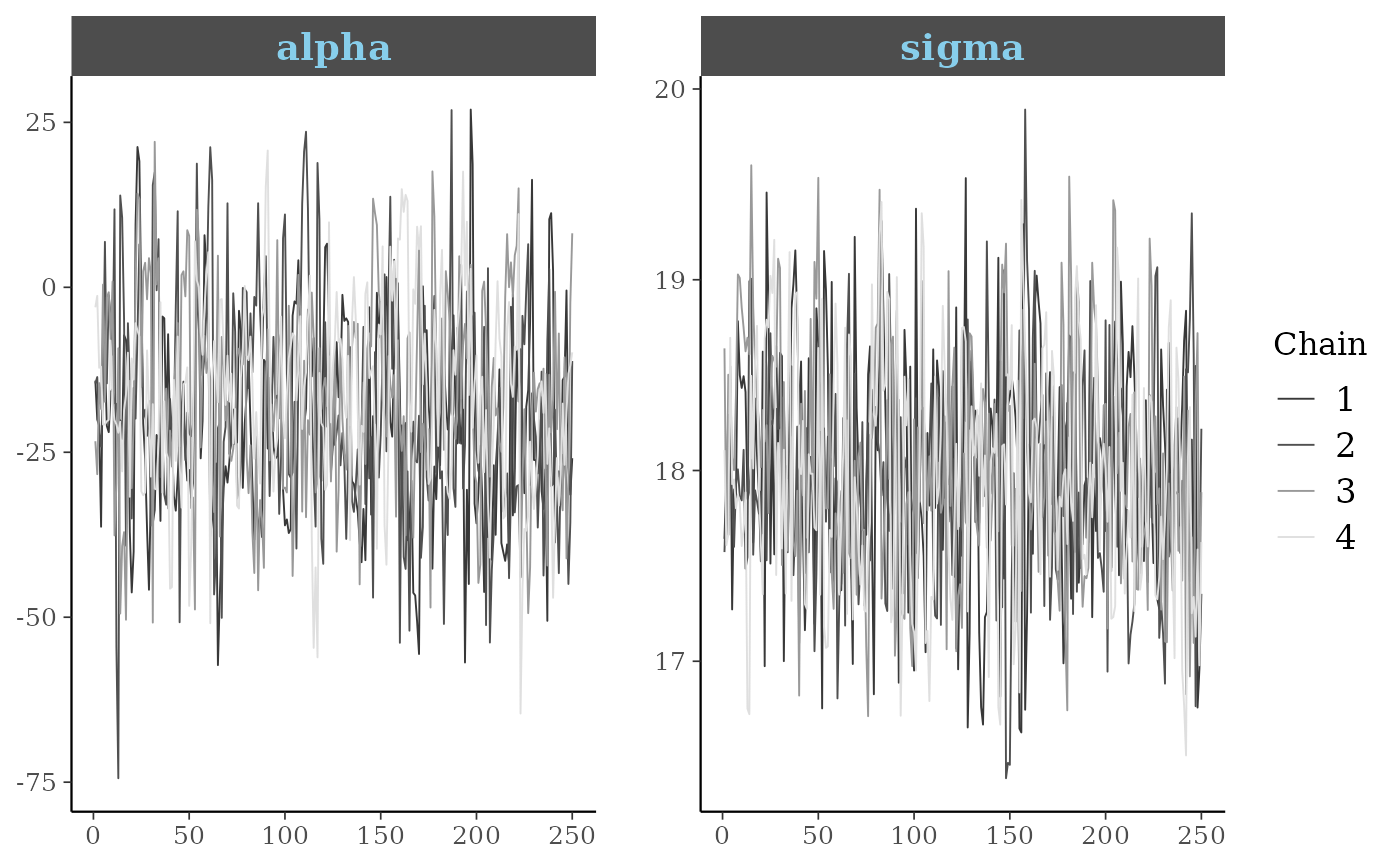# \donttest{
##########################
### control tick marks ###
##########################
p4 +
myfacets +
yaxis_text(FALSE) +
yaxis_ticks(FALSE) +
xaxis_ticks(size = 1, color = "skyblue")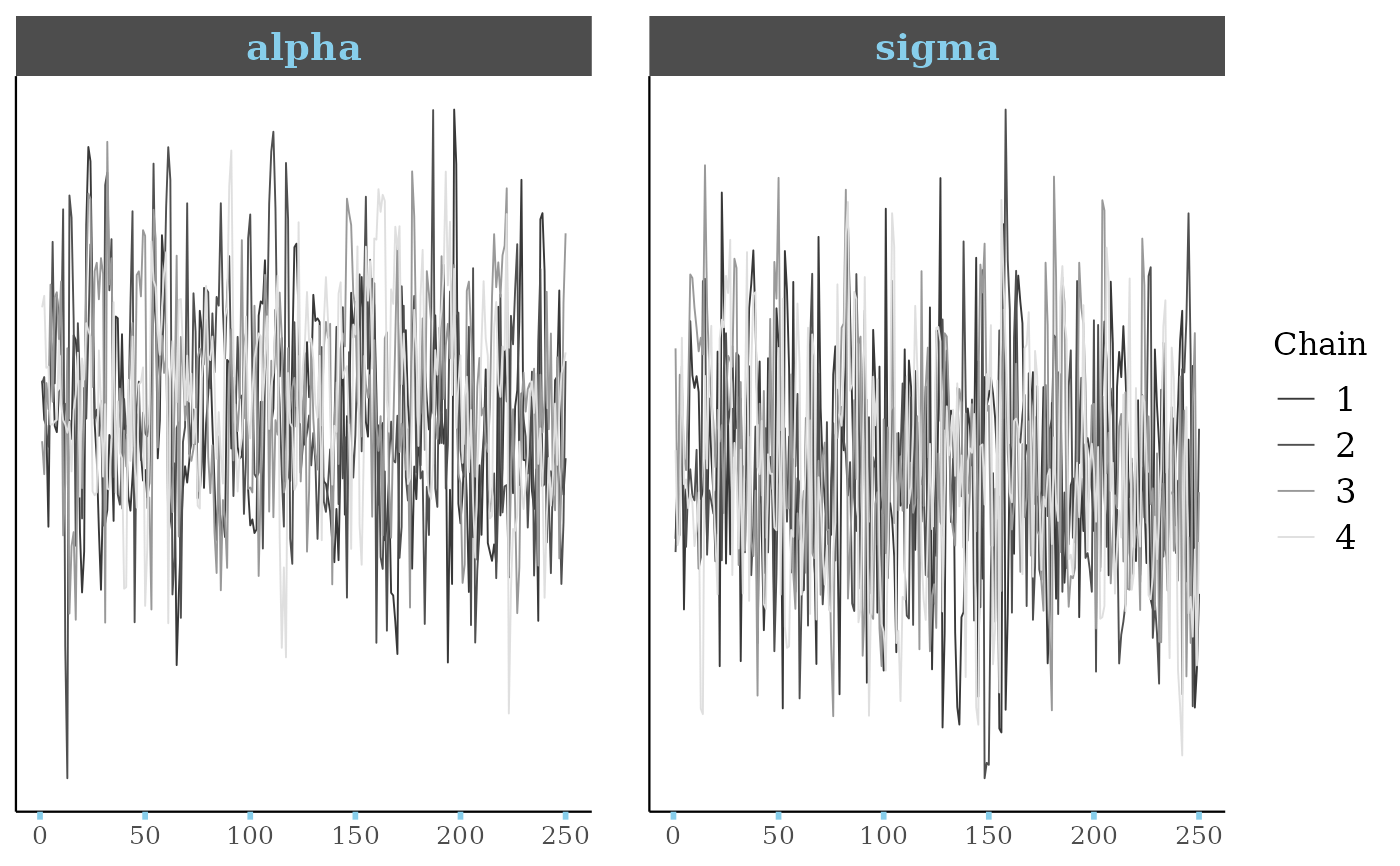# }

##############################
### change plot background ###
##############################
color_scheme_set("blue")

# add grid lines
ppc_stat(y, yrep) + grid_lines()#> stat_bin() using bins = 30. Pick better value with binwidth.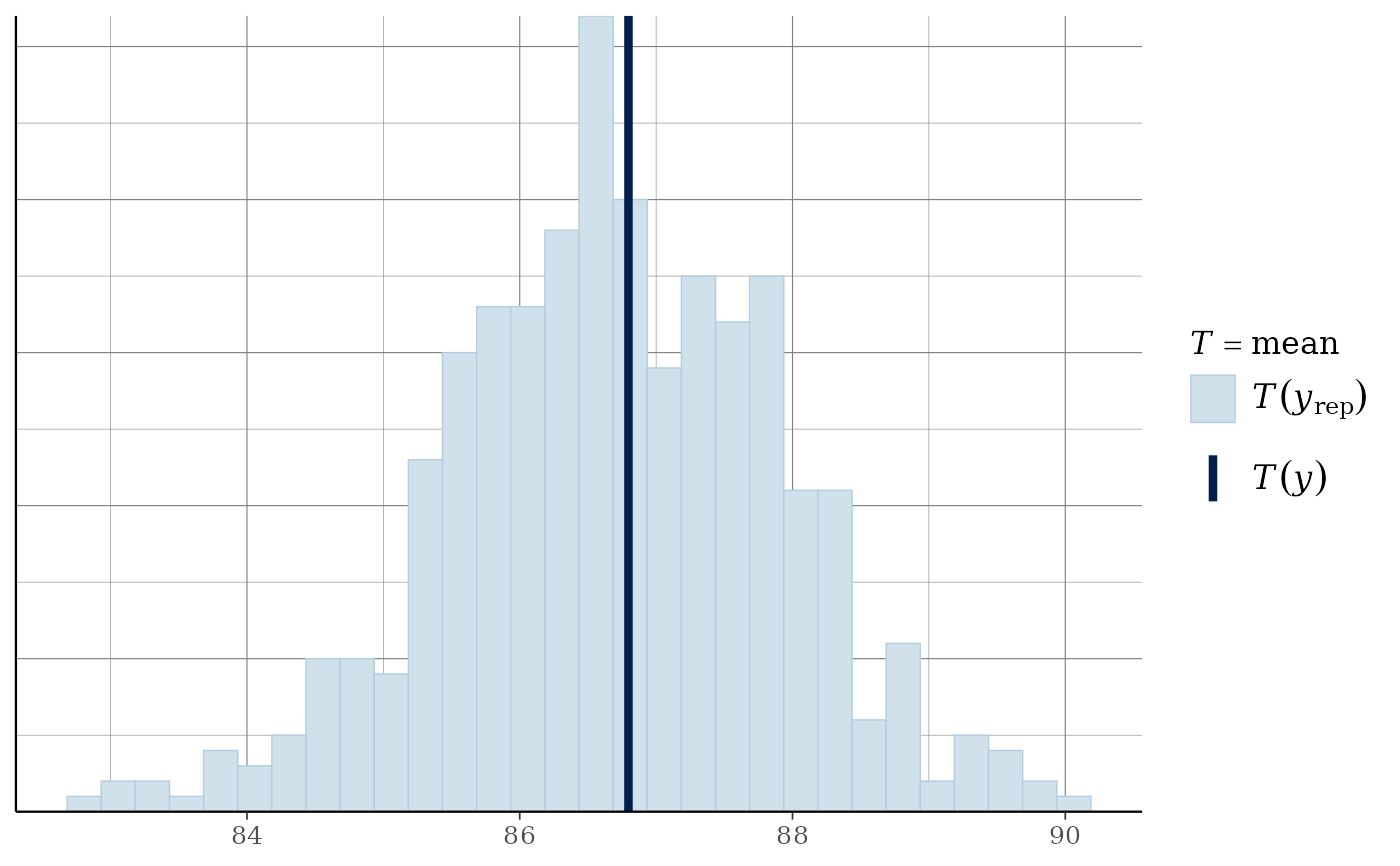# panel_bg vs plot_bg
ppc_scatter_avg(y, yrep) + panel_bg(fill = "gray90")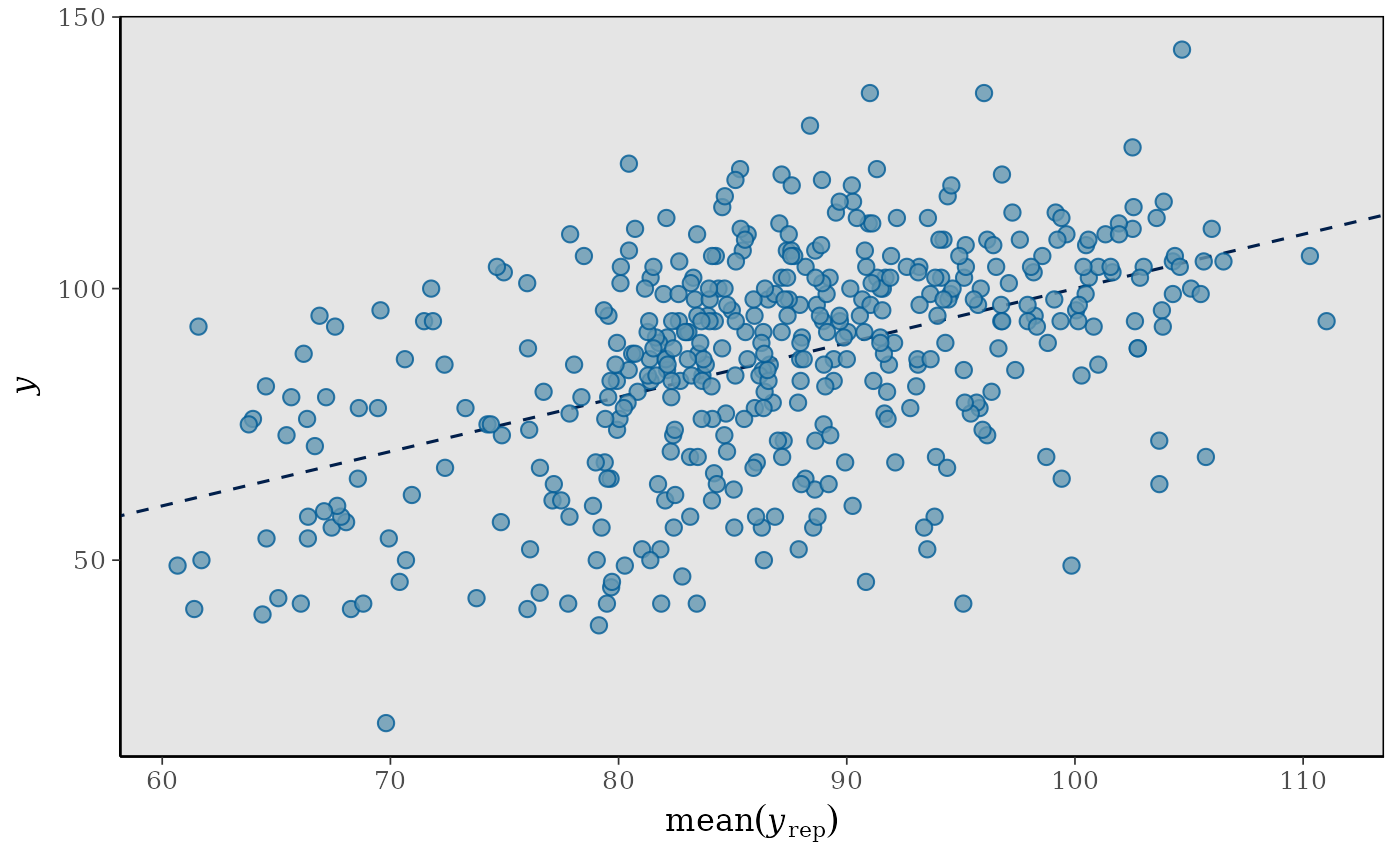ppc_scatter_avg(y, yrep) + plot_bg(fill = "gray90")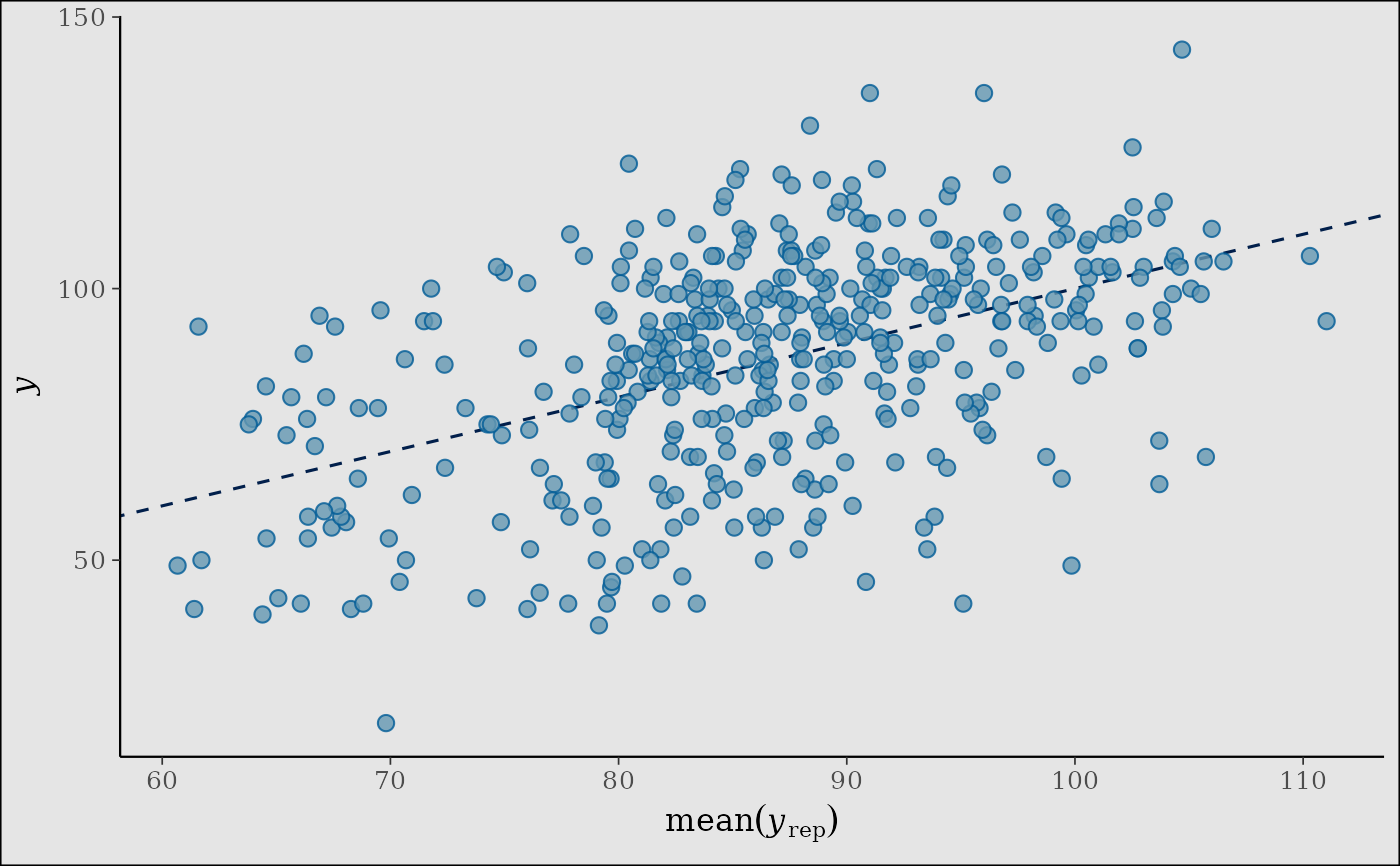color_scheme_set("yellow")
p5 <- ppc_scatter_avg(y, yrep, alpha = 1)
p5 + panel_bg(fill = "gray20") + grid_lines(color = "white")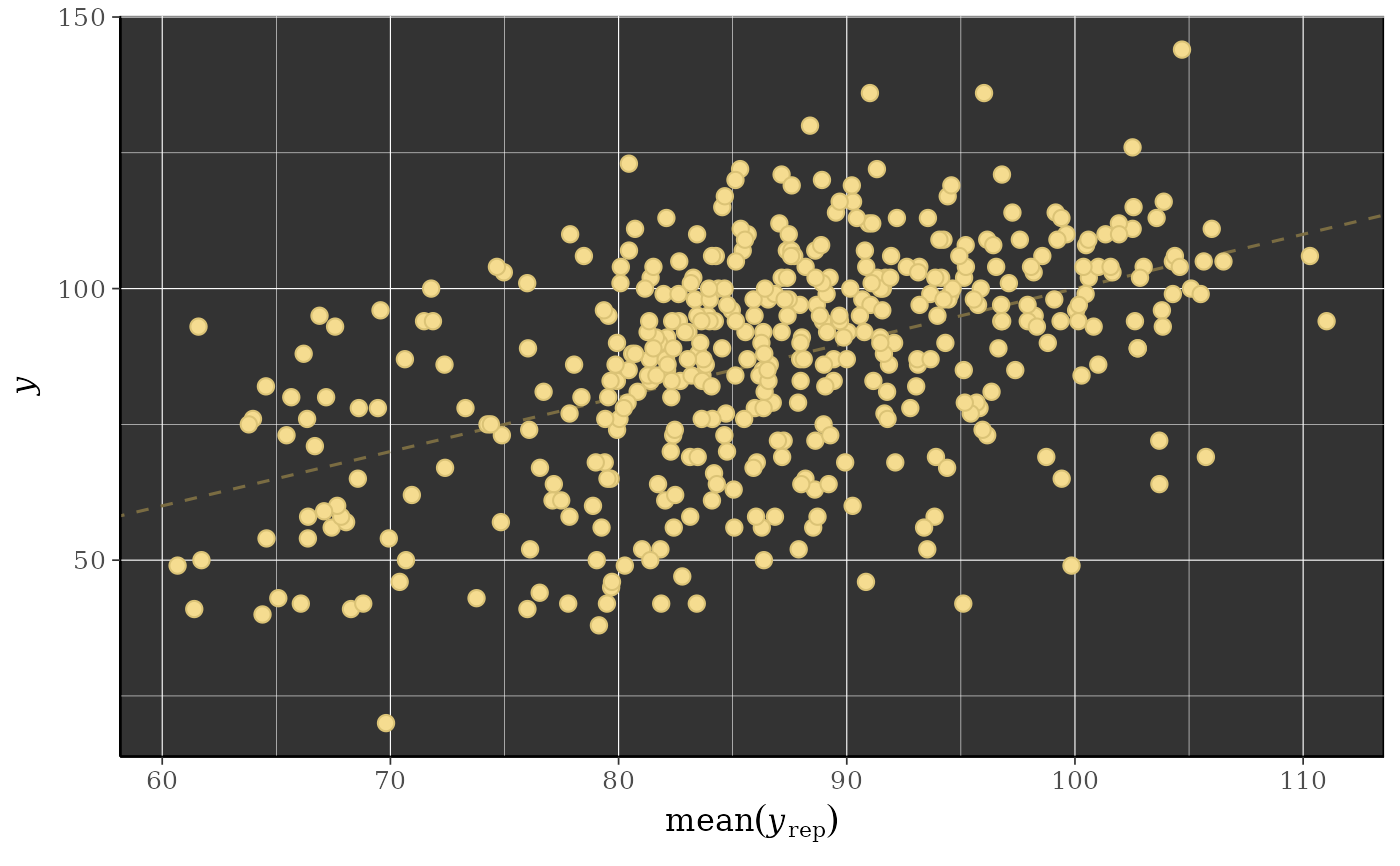# \donttest{
color_scheme_set("purple")
ppc_dens_overlay(y, yrep[1:30, ]) +
legend_text(size = 14) +
legend_move(c(0.75, 0.5)) +
plot_bg(fill = "gray90") +
panel_bg(color = "black", fill = "gray99", size = 3)# }

###############################################
### superimpose a function on existing plot ###
###############################################
# compare posterior of beta to Gaussian with same posterior mean
# and sd as beta
x <- example_mcmc_draws(chains = 4)
dim(x)#>  250   4   4purple_gaussian <-
overlay_function(
fun = dnorm,
args = list(mean(x[,, "beta"]), sd(x[,, "beta"])),
color = "purple",
size = 2
)

color_scheme_set("gray")
mcmc_hist(x, pars = "beta") + purple_gaussian#> stat_bin() using bins = 30. Pick better value with binwidth.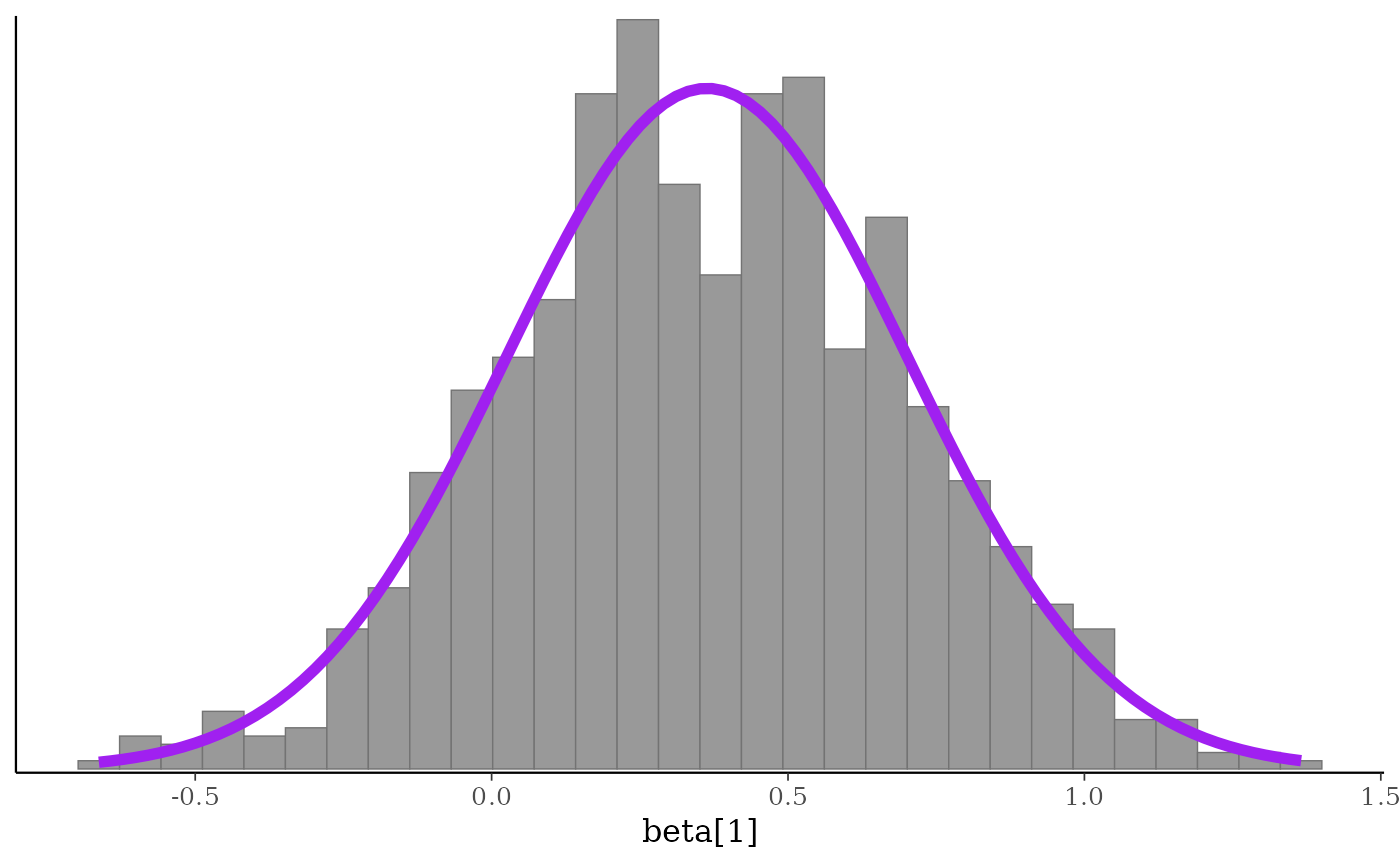mcmc_dens(x, pars = "beta") + purple_gaussian